Courses

# RD Sharma Solutions - Ex-15.3, (Part - 2), Properties Of Triangles, Class 7, Math Class 7 Notes | EduRev

## Class 7: RD Sharma Solutions - Ex-15.3, (Part - 2), Properties Of Triangles, Class 7, Math Class 7 Notes | EduRev

The document RD Sharma Solutions - Ex-15.3, (Part - 2), Properties Of Triangles, Class 7, Math Class 7 Notes | EduRev is a part of the Class 7 Course RD Sharma Solutions for Class 7 Mathematics.
All you need of Class 7 at this link: Class 7

11. In Fig, ABC is a right triangle right angled at A. D lies on BA produced and DE perpendicular to BC intersecting AC at F. If ∠AFE = 130°, find

(i) ∠BDE

(ii) ∠BCA

(iii) ∠ABC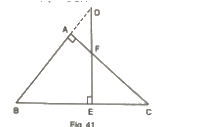Solution.

(i)

Here,

∠BAF + ∠FAD = 180° (Linear pair)

∠FAD =180°-∠BAF =180°– 90° = 90°

Also,

(ii) We know that the sum of all the angles of a triangle is 180°.

Therefore, for ΔBDE, we can say that :

∠BDE + ∠BED + ∠DBE = 180°.

∠DBE= 180°– ∠BDE ∠BED = 180°−90°−40°= 50°——(i)

Also,

∠FAD = ∠ABC + ∠ACB (Exterior angle property)

90° = 50° + ∠ACB

Or,

∠ACB = 90°– 50° = 40°

(iii) ∠ABC = ∠DBE =50° [From (i)]

12. ABC is a triangle in which ∠B=∠C and ray AX bisects the exterior angle DAC. If ∠DAX=70°. Find ∠ACB.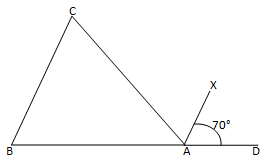Solution.

Here,

∠CAX = ∠DAX (AX bisects ∠CAD)

∠CAX =70°

∠CAX +∠DAX + ∠CAB =180°

70°+ 70° + ∠CAB =180°

∠CAB =180° –140°

∠CAB =40°

∠ACB +∠CBA + ∠CAB =180° (Sum of the angles of ΔABC)

∠ACB +∠ACB+ 40° =180° (∠C= ∠B)

2∠ACB= 180°– 40°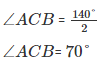13. The side BC of ΔABC is produced to a point D. The bisector of ∠A meets side BC in L. If ∠ABC=30°and ∠ACD=115°, find ∠ALC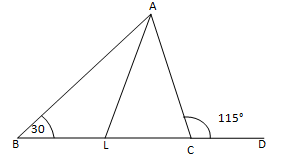Solution.

∠ACD and ∠ACL make a linear pair.

∠ACD+ ∠ACB = 180°

115° + ∠ACB =180°

∠ACB = 180°– 115°

∠ACB = 65°

We know that the sum of all angles of a triangle is 180°.

Therefore, for ΔABC, we can say that :

∠ABC + ∠BAC + ∠ACB = 180°

30° + ∠BAC + 65° = 180°

Or,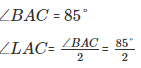Using the above rule for ΔALC, we can say that :

∠ALC + ∠LAC + ∠ACL = 180°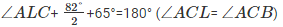Or,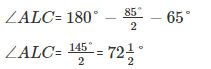14. D is a point on the side BC of ΔABC. A line PDQ through D, meets side AC in P and AB produced at Q. If ∠A = 80°, ∠ABC = 60° and ∠PDC = 15°, find

(i) ∠AQD

(ii) ∠APD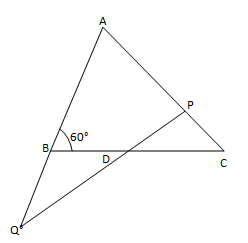Solution.

∠ABD and ∠QBD form a linear pair.

∠ABC + ∠QBC =180°

60° + ∠QBC = 180°

∠QBC = 120°

∠PDC = ∠BDQ (Vertically opposite angles)

∠BDQ = 75°

In ΔQBD :

∠QBD + ∠QDB + ∠BDQ =180° (Sum of angles of ΔQBD)

120°+ 15° + ∠BQD = 180°

∠BQD = 180°– 135°

∠BQD=45°

∠AQD = ∠BQD = 45°

In ΔAQP:

∠QAP + ∠AQP + ∠APQ = 180° (Sum of angles of ΔAQP)

80° + 45° + ∠APQ = 180°

∠APQ= 55°

∠APD = ∠APQ

15. Explain the concept of interior and exterior angles and in each of the figures given below. Find x and y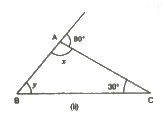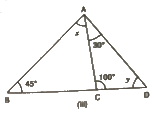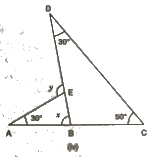Solution.

The interior angles of a triangle are the three angle elements inside the triangle.

The exterior angles are formed by extending the sides of a triangle, and if the side of a triangle is produced, the exterior angle so formed is equal to the sum of the two interior opposite angles.

Using these definitions, we will obtain the values of x and y.

(I) From the given figure, we can see that:

∠ACB + x = 180° (Linear pair)

75°+ x = 180°

Or,

x = 105°

We know that the sum of all angles of a triangle is 180°.

Therefore, for ΔABC, we can say that :

∠BAC+ ∠ABC +∠ACB = 180°

40°+y+ 75° = 180°

Or,

y = 65°

(ii)

x +80° = 180° (Linear pair)

= x = 100°

In ΔABC:

x+ y+ 30° = 180° (Angle sum property)

100° + 30° + y = 180°

= y = 50°

(iii)

We know that the sum of all angles of a triangle is 180°.

Therefore, for ΔACD, we can say that :

30° + 100° + y = 180°

Or,

y = 50°

∠ACB + 100° = 180°

∠ACB = 80° … (i)

Using the above rule for ΔACD, we can say that :

x+ 45° + 80° = 180°

= x = 55°

(iv)

We know that the sum of all angles of a triangle is 180°.

Therefore, for ΔDBC, we can say that :

30° + 50° + ∠DBC = 180°

∠DBC = 100°

x + ∠DBC = 180° (Linear pair)

x = 80°

And,

y = 30° + 80° = 110° (Exterior angle property)

16. Compute the value of x in each of the following figures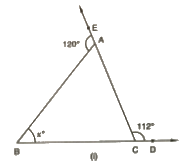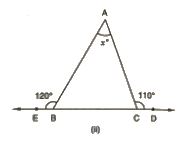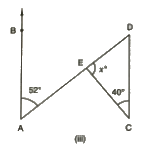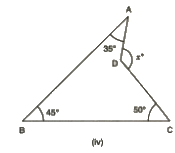Solution.

(i) From the given figure, we can say that :

∠ACD + ∠ACB = 180° (Linear pair)

Or,

∠ACB = 180°– 112° = 68°

We can also say that :

∠BAE + ∠BAC = 180° (Linear pair)

Or,

∠BAC = 180°– 120° = 60°

We know that the sum of all angles of a triangle is 180°.

Therefore, for ΔABC:

x+ ∠BAC + ∠ACB = 180°

x = 180°– 60°– 68° = 52°

= x = 52°

(ii) From the given figure, we can say that :

∠ABC + 120° = 180° (Linear pair)

∠ABC = 60°

We can also say that :

∠ACB+ 110° = 180° (Linear pair)

∠ACB = 70°

We know that the sum of all angles of a triangle is 180°.

Therefore, for ΔABC :

x+ ∠ABC + ∠ACB = 180°

= x = 50°

(iii)

From the given figure, we can see that :

We know that the sum of all the angles of a triangle is 180°.

Therefore, for ΔDEC:

x + 40°+ 52° = 180°

= x = 88°

(iv) In the given figure, we have a quadrilateral whose sum of all angles is 360°.

Thus,

35° + 45° + 50° + reflex∠ADC = 360°

Or,

230° + x = 360° (A complete angle)

= x = 130°

The document RD Sharma Solutions - Ex-15.3, (Part - 2), Properties Of Triangles, Class 7, Math Class 7 Notes | EduRev is a part of the Class 7 Course RD Sharma Solutions for Class 7 Mathematics.
All you need of Class 7 at this link: Class 7Use Code STAYHOME200 and get INR 200 additional OFF Use Coupon Code
All Tests, Videos & Notes of Class 7: Class 7

### Up next### Top Courses for Class 7## RD Sharma Solutions for Class 7 Mathematics

97 docs

### Up next### Top Courses for Class 7Track your progress, build streaks, highlight & save important lessons and more!

,

,

,

,

,

,

,

,

,

,

,

,

,

,

,

,

,

,

,

,

,

,

,

,

,

,

,

,

,

,

,

,

,

;